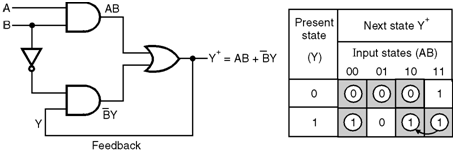Home > finite state machines > Hazards > Effects of Hazards

# Effects of Hazards :

• The effect of seriousness of hazards is dependent on the devices or systems which receive the momentary transient signals.
• In case of asynchronous sequential networks, the presence of hazards can cause the network to malfunction.
• Consider the network shown in Figure below. The Y output is connected as one of the inputs. So feedback is existing in this network.
• The excitation table for this circuit has been shown in Figure. The stable states have been circled.
• The excitation table indicates that the total input state (A, B, Y) = (11, 1) corresponds to a stable condition . If input B is changed from 1 to 0, then the operating point moves horizontally to another stable total input state (A B Y) = (10, 1) as shown by the arrow in Figure..
• But the given circuit in Fig. 2.13.1(a) is same as the one used in Figure (illustration of static 1 hazard). So there is a possibility of static 1 hazard when input B changes.
• Due to static 1 hazard, the output Y will go 0 momentarily, which is fed back to gate G2 in Figure.(a) Given circuit (b) Excitation table
• If the delay of NOT gate is appreciably long then, this momentary â€œ0â€ at Y will enter into gate G2 before the NOT gate making a transition from 0 to 1, then the net result is that the network becomes stable with the total input state (A, B, Y) = (10, 0).
• Thus the network becomes stable, in an undesired state. So we conclude that the presence of static 1 hazard has made the network to malfunction.